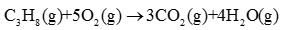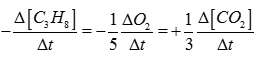# Chemical Kinetics - Online Test

Q1. Study of rate of chemical reaction is known as
Explaination / Solution:

Chemical Kinetics deals with rate of a Chemical reactions.

Q2. Which among the following is an instantaneous reaction?
Explaination / Solution:

Precipitation of AgCl is an example of instantaneous reaction.

Q3. The rate of reaction can be measured as
Explaination / Solution:

Rate of reaction can be measured in terms of Rate of disappearance of reactant or rate of apperance of product.

Q4. Rate of reaction for the combustion of propane is equal toExplaination / Solution:Q5.
What is the order of a reaction which has a rate expression ; Rate =
Explaination / Solution:

Total order of reaction = order wrt to A + order wrt to B.

Q6. Molecularity of a reaction
Explaination / Solution:

Molecularity means minimum no. of atoms molecules or ions which undergo collision for conversion of reactant to Product. So it is a whole no. Cannot be fractional or negative.

Q7. For which of the following reaction the units of rate constant and rate of the reaction are same?
Explaination / Solution:

Rate=k[Reactant]0
Q8. Which of the following statement is true for order of a reaction?
Explaination / Solution:

Order of a reaction is experimentally determined quantity so it can be negative fractional or zero.

Q9.
For a reaction; pA +qB products, the rate law expression is
Explaination / Solution:

Order of a reaction is experimentally determined quantity. It may or may not be equal to stoichiometric coefficients.

Q10. For the first order reaction, half life is equal to
Explaination / Solution:

For 1st order reaction half life is independent of initial concentration of reactant.